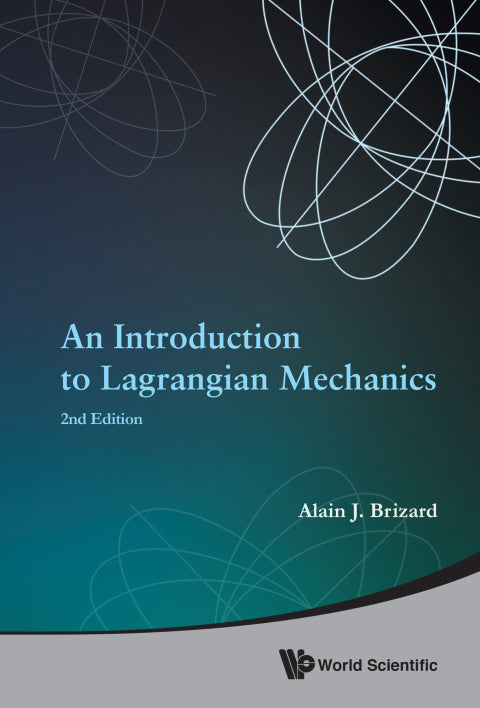• Author(s) Alain J Brizard
• Edition
• Published11282014
• PublisherWorld Scientific Publishing
• ISBN9789814623643
An Introduction to Lagrangian Mechanics begins with a proper historical perspective on the Lagrangian method by presenting Fermat's Principle of Least Time (as an introduction to the Calculus of Variations) as well as the principles of Maupertuis, Jacobi, and d'Alembert that preceded Hamilton's formulation of the Principle of Least Action, from which the Euler-Lagrange equations of motion are derived. Other additional topics not traditionally presented in undergraduate textbooks include the treatment of constraint forces in Lagrangian Mechanics; Routh's procedure for Lagrangian systems with symmetries; the art of numerical analysis for physical systems; variational formulations for several continuous Lagrangian systems; an introduction to elliptic functions with applications in Classical Mechanics; and Noncanonical Hamiltonian Mechanics and perturbation theory.The Second Edition includes a larger selection of examples and problems (with hints) in each chapter and continues the strong emphasis of the First Edition on the development and application of mathematical methods (mostly calculus) to the solution of problems in Classical Mechanics.New material has been added to most chapters. For example, a new derivation of the Noether theorem for discrete Lagrangian systems is given and a modified Rutherford scattering problem is solved exactly to show that the total scattering cross section associated with a confined potential (i.e., which vanishes beyond a certain radius) yields the hard-sphere result. The Frenet-Serret formulas for the Coriolis-corrected projectile motion are presented, where the Frenet-Serret torsion is shown to be directly related to the Coriolis deflection, and a new treatment of the sleeping-top problem is given.

# Introduction To Lagrangian Mechanics, An (2nd Edition)

##### Get it instantly

Zookal account needed

\$71.08 \$74.79 Save \$3.71
• Author(s) Alain J Brizard
• Edition
• Published11282014
• PublisherWorld Scientific Publishing
• ISBN9789814623643
An Introduction to Lagrangian Mechanics begins with a proper historical perspective on the Lagrangian method by presenting Fermat's Principle of Least Time (as an introduction to the Calculus of Variations) as well as the principles of Maupertuis, Jacobi, and d'Alembert that preceded Hamilton's formulation of the Principle of Least Action, from which the Euler-Lagrange equations of motion are derived. Other additional topics not traditionally presented in undergraduate textbooks include the treatment of constraint forces in Lagrangian Mechanics; Routh's procedure for Lagrangian systems with symmetries; the art of numerical analysis for physical systems; variational formulations for several continuous Lagrangian systems; an introduction to elliptic functions with applications in Classical Mechanics; and Noncanonical Hamiltonian Mechanics and perturbation theory.The Second Edition includes a larger selection of examples and problems (with hints) in each chapter and continues the strong emphasis of the First Edition on the development and application of mathematical methods (mostly calculus) to the solution of problems in Classical Mechanics.New material has been added to most chapters. For example, a new derivation of the Noether theorem for discrete Lagrangian systems is given and a modified Rutherford scattering problem is solved exactly to show that the total scattering cross section associated with a confined potential (i.e., which vanishes beyond a certain radius) yields the hard-sphere result. The Frenet-Serret formulas for the Coriolis-corrected projectile motion are presented, where the Frenet-Serret torsion is shown to be directly related to the Coriolis deflection, and a new treatment of the sleeping-top problem is given.